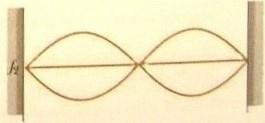# Problem: The figure shows a standing wave on a string.Suppose the tension is doubled while the frequency shaking the string is held constant. Will there be a standing wave? If so how many antinodes will it have? If not, why not?

###### FREE Expert Solution

Frequency of oscillation for a stretched string:

$\overline{){\mathbf{f}}{\mathbf{=}}\frac{\mathbf{n}}{\mathbf{2}\mathbf{L}}{\mathbf{·}}\sqrt{\frac{\mathbf{T}}{\mathbf{\mu }}}}$

Solving for n:

$\overline{){\mathbf{n}}{\mathbf{=}}{\mathbf{2}}{\mathbf{L}}{\mathbf{f}}{\mathbf{·}}\sqrt{\frac{\mathbf{\mu }}{\mathbf{T}}}}$

The initial nodes, ni = 2, and initial tension, Ti.

The final tension is 2Ti.

We'll solve for new nodes, nfinal, as follows:

${\mathbit{n}}_{\mathbf{i}}\mathbf{=}\mathbf{2}\mathbit{L}{\mathbit{f}}_{\mathbf{1}}\mathbf{·}\sqrt{\frac{\mathbf{\mu }}{{\mathbf{T}}_{\mathbf{i}}}}$

${\mathbit{n}}_{\mathbf{f}}\mathbf{=}\mathbf{2}\mathbit{L}{\mathbit{f}}_{\mathbf{1}}\mathbf{·}\sqrt{\frac{\mathbf{\mu }}{\mathbf{2}{\mathbf{T}}_{\mathbf{i}}}}$

94% (74 ratings)###### Problem DetailsThe figure shows a standing wave on a string.

Suppose the tension is doubled while the frequency shaking the string is held constant. Will there be a standing wave? If so how many antinodes will it have? If not, why not?